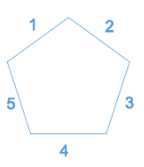Common Core: 2nd Grade Math : Recognize and Draw Shapes: CCSS.Math.Content.2.G.A.1

Example Questions

← Previous 1 3

Example Question #26 : How To Find The Perimeter Of A Triangle

Which shape has onlysides?

Pentagon

Hexagon

Square

Cube

Triangle

Triangle

Explanation:

A triangle hassides.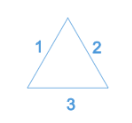Example Question #39 : How To Find The Perimeter Of A Square

Which shape has onlyangles?

Cube

Square

Pentagon

Hexagon

Triangle

Square

Explanation:

A square as onlyangles.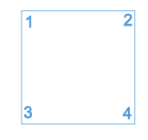Example Question #20 : How To Find The Perimeter Of A Square

Which shape has onlysides?

Cube

Triangle

Square

Hexagon

Pentagon

Square

Explanation:

A square hassides.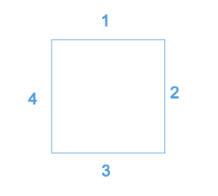Example Question #21 : How To Find The Perimeter Of A Square

Which shape has onlyangles?

Cube

Hexagon

Triangle

Square

Pentagon

Pentagon

Explanation:

A pentagon hasangles.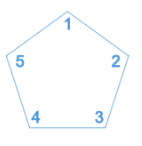Example Question #22 : How To Find The Perimeter Of A Square

Which shape has onlysides?

Triangle

Square

Pentagon

Cube

Hexagon

Hexagon

Explanation:

A hexagon hassides.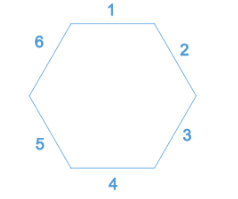Example Question #23 : How To Find The Perimeter Of A Square

Which shape has onlyangles?

Cube

Pentagon

Triangle

Hexagon

Explanation:

Quadrilaterals are shapes withsides andangles.

Example Question #24 : How To Find The Perimeter Of A Square

Which shape has onlysides?

Cube

Triangle

Pentagon

Hexagon

Explanation:

Quadrilaterals are shapes withsides andangles.

Example Question #25 : How To Find The Perimeter Of A Square

Which shape hasfaces?

Pentagon

Square

Cube

Triangle

Hexagon

Cube

Explanation:

A cube has 6 faces.

Example Question #1 : Geometry

Which shape hasangles?

Pentagon

Hexagon

Triangle

Cube

Rectangle

Rectangle

Explanation:

A rectangle hasangles.Example Question #27 : How To Find The Perimeter Of A Square

What shape has onlysides?

Triangle

Square

Cube

Pentagon

Hexagon

A pentagon hassides.